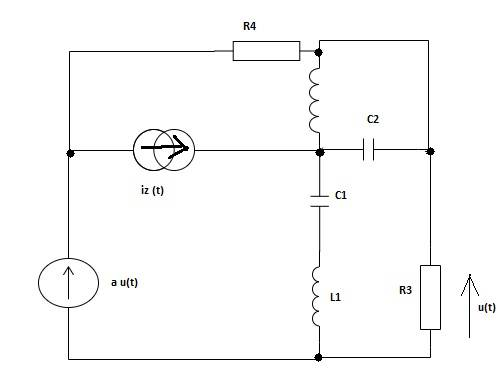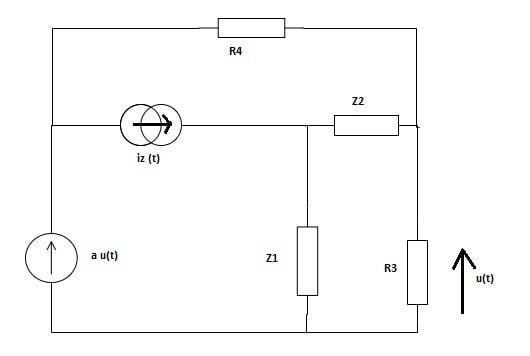# AC - Node Voltage Method for difficult circuit

• devmew
The first equation is the voltage gain of the first circuit. The second equation is the voltage loss of the first circuit. The third equation is the voltage gain of the second circuit. The fourth equation is the voltage loss of the second circuit.

#### devmew

Hi!
I'm trying to understand and solve this circuit by Node Voltage Method. I have to calculate u(t). In my mind, I don't have any idea if any current should go through Z1 in my calculations.
I would be grateful if you could write even equations for this circuit. Is 'a' just a prefix to multiply the value of voltage source? (z is the letter for 'complex' in my language).

Data:
iz(t)=2cos(2t−45°) A C1=1/2 [F] C2=1/3 [F] L1=1[H] L2=3[H]
R3=2[Ω] R4=1[Ω] a=1/2 [V/V]My calculations:

Welcome to PhysicsForums.Is a*u(t) a VCVS? That seems like a non-standard voltage source symbol, but it does look like you are using a different symbol for the iz(t) source...

Also, I don't see how the 2nd circuit follows from the first, but I could be missing something. Were you given the 1st circuit and you wanted to simplify it via the 2nd diagram?

•devmew
a=e^(-j*2*π/3)=cos(-2*π/3)+jsin(-2*π/3)

@berkeman a*u(t) is only hint, needed to calculations. It describes the relationship between voltage source and voltage on resistor. 'a' is just a coefficient. I was given the first circuit and I wanted to simplify it via 2nd diagram.
@Babadag I'm looking to get the value u(t) in function of sinx. So, I changed it into sin function.
$$i_z(t)=2cos(2t-45°)=2sin(2t-45°+90°)=2sin(2t+45°)$$
$$I_z=\frac{2}{\sqrt{2}}(cos(45°)+j sin(45°))=\frac{2}{\sqrt{2}}(\frac{\sqrt{2}}{2}+j\frac{\sqrt{2}}{2})=1+j$$

a*u(t) is the voltage source, and iz(t) is the power source. I'm sorry, I didn't know the worldwide symbols.

$$Z_1=\frac{1}{jωC_1}+jωL_1=\frac{1}{j}+2j=-j+2j=j$$
$$\frac{1}{Z_2}=\frac{1}{jωL_2}+jωC_2$$
$$Z_2=\frac{6}{3j}=-2j$$

Last edited: